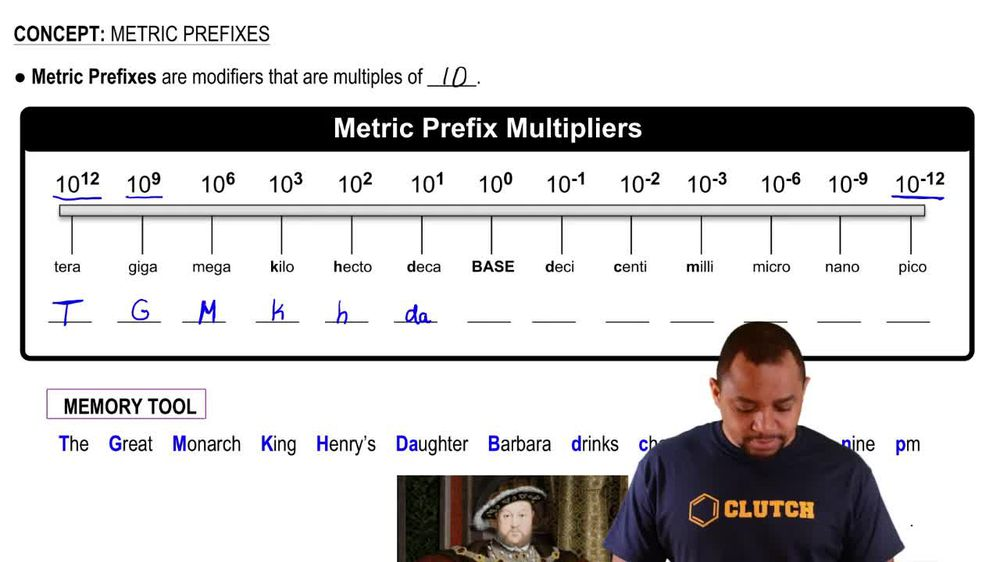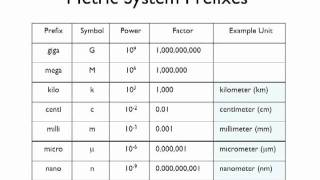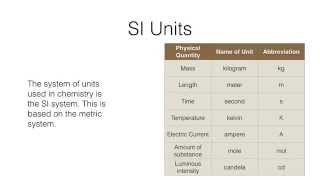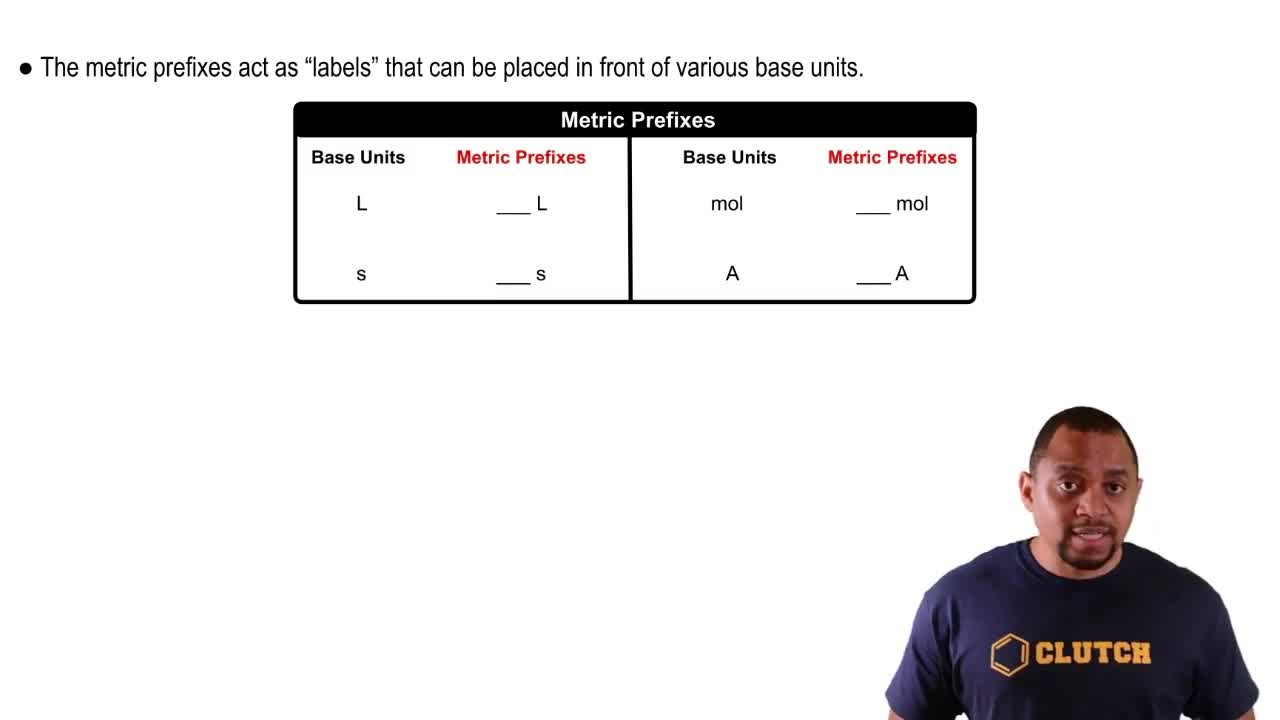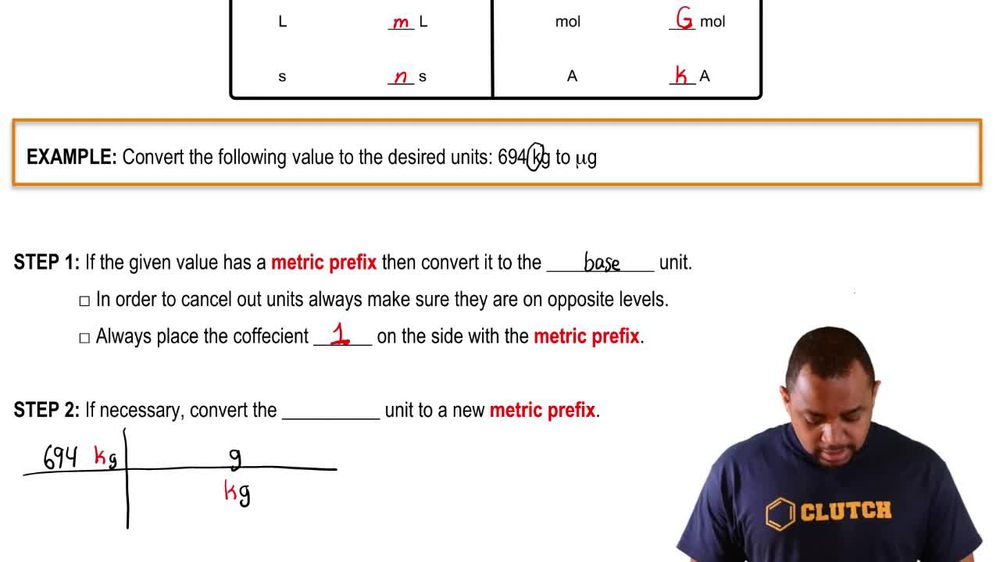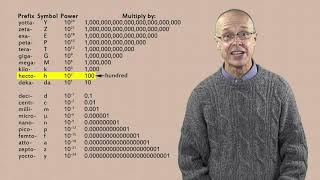Start typing, then use the up and down arrows to select an option from the list.
1. 1. Intro to General Chemistry2. Metric Prefixes
Problem

# The radius of an atom of copper (Cu) is about 140 pm. (a) Express this distance in angstroms 1A 2.

Relevant Solution57s
Play a video:
Hi everyone today, we have a question asking us to express the following using base units and scientific notation, and our Value is 452 Mem. So our base unit there is going to be meters, so one mega meter Equals 10 to the 6th m. So we have 452 mega meters And we're going to multiply that by 10-6 m and one mega meter. So our mega meter is going to cancel out here, And that is going to give us 4. Times, 10 to the 8th m. And that is our final answer. Thank you for watching. Bye.